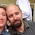## The Leaf/Grid Question

So we got together with the class next door - @CapitanoAmazing and 4RB -  to explore a bit of area of 2D shapes.

We were using the old "find the area of the leaf on the the grid" question as a starting point - a bit of tuning in to get some ideas going.

## What about the wonky bits?

The clever students in Year 4 quickly observed that the leaf diagrams didn't fit onto the grid very well. There were a few whole squares but most of the squares were only part squares. What do you do with the wonky bits?

We came up with 3 suggestions:

1. Pairing up - pair up each part square with another that is about the size that combined they will make a whole square.

2. Wonky square formula - every part square, regardless of its size, is worth half a full square, or ½ cm2.

3. Fraction addition - approximate what fraction each part square is and then add these fractions together - good luck with this one guys!

## Get Real!

Of course, if we're doing maths then we need to use something real - like why do we need a picture of a leaf when we can go and get a real one?

And once we got some real leaves, the interesting questions started flowing...

## If I move my leaf to a different place on the grid, does the area change?

One young man got himself a very small leaf - less than 1cm2

Depending on where he places this leaf on the grid and also depending on which method of estimation he used, he got different answers.

Did the area of the leaf change? - No of course not.

So why were there different answers?

The kids worked out that the systems they were using were only estimations - useful for finding the areas of irregular shapes.

## But how do we make it more accurate?

One boy suggested that it would be more accurate to use percentages to calculate the area of the part squares. And it would be easier than adding a whole bunch of fractions.

And then one of the girls suggested....

"These are 1cm squares. If we divided each square into a 10mm x 10mm grid..."

#### 1 comment:

1.Great project Bruce. I've thought about a way you could extend this into a calculus project. If students modelled the edge of the leaf using functions they know, they could then find the area using integration.

Thank you for sharing it. I'm going to use this project with my calculus students later this school year.

Any comments you would like to make?